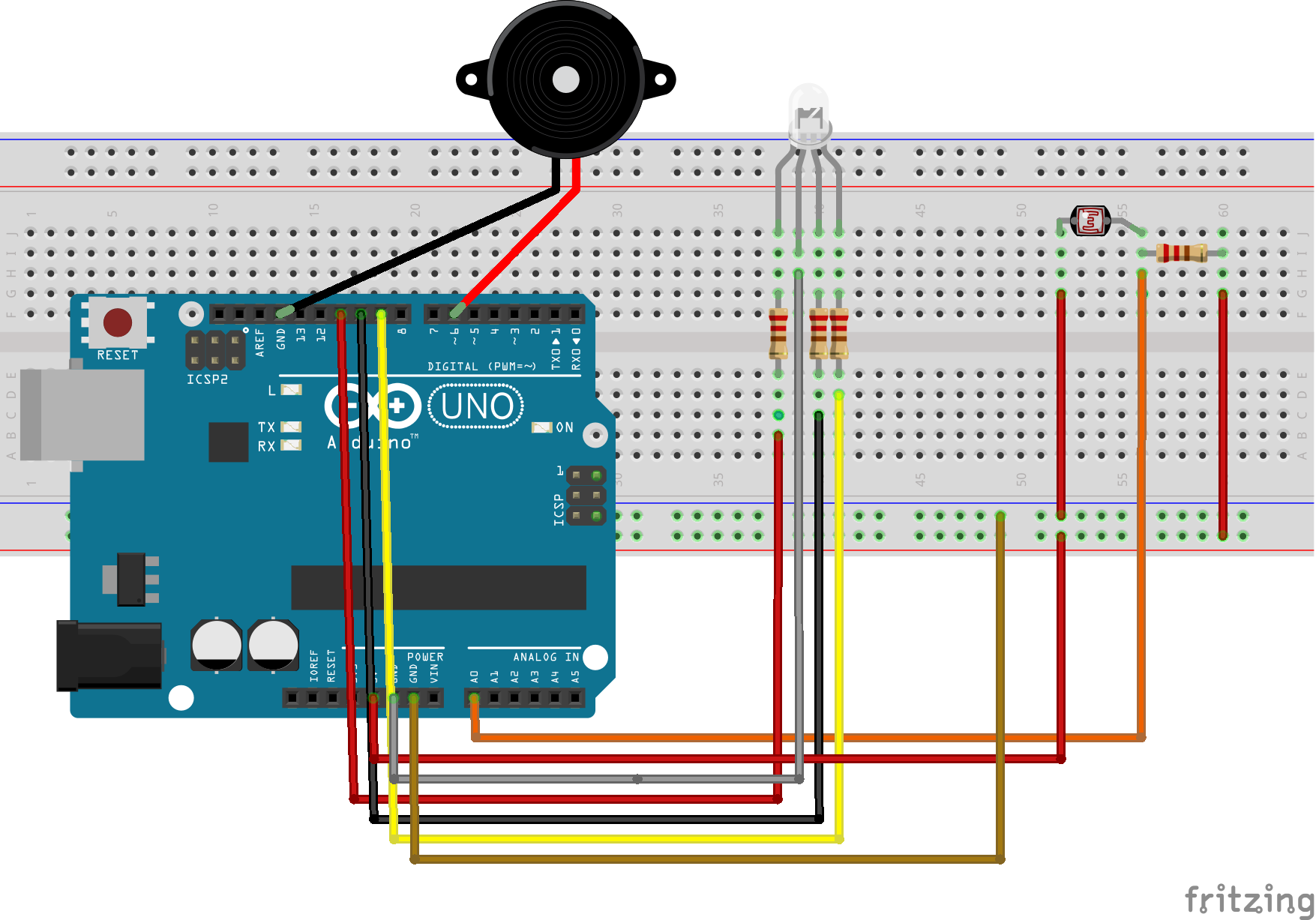### LED RBG and buzzer controlled by LDR

My final project for the 2nd term is controlling the color of the RGB LED, and the frequency of the buzzer, depending on the light recieved by the LDR. The hardware I have used are written down:

• Arduino Leonardo or Uno(I've tried both)
• Buzzer
• LED RGB
• LDR
• Wires
• 330Ω and 7k6Ω resistors

And here is the scheme of the circuit, made with fritzing:Down you have the code I created:

``` //LDR controlling RGB LED and buzzer int valueLDR, freq; int ledRed=9; int ledGreen=10; int ledBlue=11; int pinLDR=A0; int speaker=6; void setup() { // put your setup code here, to run once: pinMode(ledRed, OUTPUT); pinMode(ledGreen, OUTPUT); pinMode(ledBlue, OUTPUT); //analogReference(EXTERNAL); Serial.begin(9600); } void loop() { Serial.println(valueLDR); delay(1); // put your main code here, to run repeatedly: valueLDR=analogRead(pinLDR); int Red = map(valueLDR,50,500,0,255); int Green = map(valueLDR,50,500,255,0); int Blue = map(valueLDR,50,500,0,128); int freq = map(valueLDR,50,500,261,494); analogWrite(ledRed,Red); analogWrite(ledGreen,Green); analogWrite(ledBlue,Blue); tone(speaker,freq,10); } ```

On that code, I defined the variables as an integer number, and they are connected as an analog output. That code lets you know also the quantity of light that is receiving the LDR (between 0-1023). This is compoulsary if you want to work as much accurately as possible. I took the information given by the monitor serie to adjust the mapped. What I did with the map is changing the scale(which they can oscillate depending on the conditions of light you are submitted, and also the resistor you are using), from the highest and lowest values given before to 0-255.

##### Difficulties

The main difficulty I had while doing that project was joining both circuts, the LDR and RGB ones, in order to work well both, but they didn't work well together at first. So the first thing I tried was making a simple traffic light circuit, just to make sure the lights worked. You can visit that simple project by clicking here: Traffic lights.

Once I had proved the lights were OK, I contiuned by checking the LDR. I used this simple code:

``` void setup () { Serial.begin(9600); } void loop() { int sensorvalue=analogRead(A0); Serial.println(sensorvalue); delay(1); } ```

This code gives you the quantity of light(0-1023) of light recieved by the LDR(defined as sensor value and connected to A0), each millisecond, by clicking on "Monitor serie" Here you have some videos of the process with difference types of resistors to notice the difference between them.

This process let me know which were the best values to map between. Nevertheless, they weren't working if I put them together. It started upsetting, when we realized the code we devoloped our from, had a potenciometer on the circuit, but we did not. So it was just about deleting that line:

`analogReference(EXTERNAL);`

##### Extension

Once we had finished the project, we decided to put a multimeter, to mesure the total resistence of the circuit with diferent resistors in order to know which was the best to use. Below you have the results:

330Ω±5%:

• LDR uncovered:The total resistence was 1078 Ω, the LED was purple, and the monitor serie marked 860
• LDR covered:The total resistence was 1076 Ω, the LED was green, and the monitor serie marked 970
• LDR dazzled:The total resistence was 1075 Ω, the LED was purple, and the monitor serie marked 140

10000Ω±5%:

• LDR uncovered:The total resistence was 51700 Ω, the LED was a green , and the monitor serie marked 115
• LDR covered:The total resistence was 51600 Ω, the LED was clear purple, and the monitor serie marked 270
• LDR dazzled:The total resistence was 51700 Ω, the LED was purple, and the monitor serie marked 4

22000Ω±5%:

• LDR uncovered:The total resistence was 51800 Ω, the LED was green, and the monitor serie marked 70
• LDR covered:The total resistence was 51700 Ω, the LED was purple, and the monitor serie marked 300
• LDR dazzled:The total resistence was 51600 Ω, the LED was purple, and the monitor serie marked 6

In conclusion, the greater resistor was the first one, 330Ω±5%. That's simply because if we put it on the circuit, the LDR will be able to mark larger variety of numbers than the rest of the resistors.